Daily Practice Problems
Class 10 Maths
Pair of Linear Equations in Two VariablesQuestion 1:

Solve 2x + 3y = 11 and 2x – 4y = – 24 and hence find the value of ‘m’ for which y = mx + 3.

Question 2:

Find whether the given pair of linear equations is consistent or inconsistent.

3x + 2y = 8, 6x – 4y = 9

Question 3:

A motor boat can travel 30 km upstream and 28 km downstream in 7 hours. It can travel 21 km upstream and return in 5 hours. Find the speed of boat in still water and the speed of stream.

Question 4:

The sum of the digits of a two digit number is 8 and the difference between the number and that formed by reversing the digits is 18. Find the number.

Question 5:

A train covered a certain distance at a uniform speed. If the train would have been 10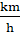faster, it would have taken 2 hours less than the scheduled time. And, if the train were slower by 10; it would have taken 3 hours more than the scheduled time. Find the distance covered by the train.

Question 6:

The age of the father is twice the sum of the ages of his 2 children. After 20 years, his age will be equal to the sum of the ages of his children. Find the age of the father.

Question 7:

Find the value of k for which the system kx + 2y = 5 and 3x + y = 1 has

(i) a unique solution                                                   (ii) no solution

Question 8:

7x – 5y – 4 = 0 is given. Write another linear equation, so that the lines represented by the pair are:

(i) intersecting                            (ii) coincident                       (iii) parallel

Question 9:

Solve the following pair of linear equations:

8x + 5y = 9

3x + 2y = 4

Question 10:

Solve: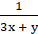+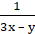=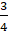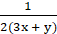-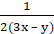=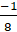Question 11:

If the lines given by 3x + 2ky = 2 and 2x + 5y + 1 = 0 are parallel, then find value of k.

Question 12:

Write the number of solutions of the following pair of linear equations:

x + 2y – 8 = 0, 2x + 4y = 16

Question 13:

If x = a, y = b is the solution of the pair of equation x – y = 2 and x + y = 4, then find the value of a and b.

Question 14:

In ∆ABC, A = x, B = 3x, and C = y if 3y – 5x = 30°, show that triangle is right angled.

Question 15:

Half the perimeter of a rectangle garden, whose length is 4 m more than its width, is 36 m. Find the dimensions of the garden.

Question 16:

The solution of 4x+ 3y = 14 and 3x- 4y = 23 is ______.

Question 17:

If a pair of linear equations is consistent, then the lines will be _____.

Question 18:

Check whether the pair of equations

x + 3y = 6

2x – 3y = 12

is consistent. If so, solve them graphically

Question 19:

The graph of x = 2 is a line parallel to ____ axis.

Question 20:

How many solutions does the pair of equations y = 0 and y = -5 have?

**********